1.梯度下降法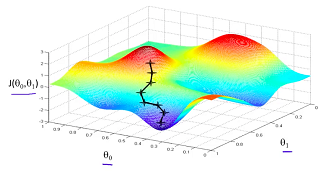1. 给定初始值$$x_0$$。
2. 按照如下方式“下山”：$$x_{i+1} = x_i-\eta\nabla_{x_i} f$$ 。其中$$\eta>0$$，在机器学习领域，$$\eta$$也被称之为学习率(learning rate)。
3. 直到$$x$$ 满足收敛条件为止。如$$\|f(x_{i+1}) – f(x_i)\|<\epsilon$$或$$||\nabla_{x_i}f||\approx 0$$。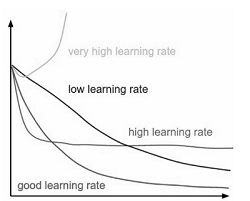1.1 梯度下降法的优势

1.时间复杂度低，在每一个迭代中，只需要计算梯度，不需要对二阶导数矩阵（即海森矩阵(Hessian Matrix)）进行计算。

2.空间复杂度低，因为梯度向量为一个$$n\times 1$$的向量，比起Hessian Matrix来，占用存储空间小n倍。在实际应用中，$$\mathbf{x}$$的维度可能非常高。

1.2梯度下降法的局限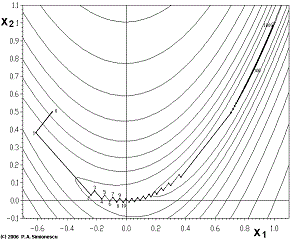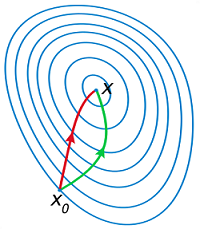1.3 最速下降法(steepest decent) 与  梯度下降法(gradient descent)的联系

$$\bigtriangleup \epsilon_{nsd}=argmin_v(\nabla f(x)^T\epsilon\mid \|\epsilon\|=1)$$

2.牛顿法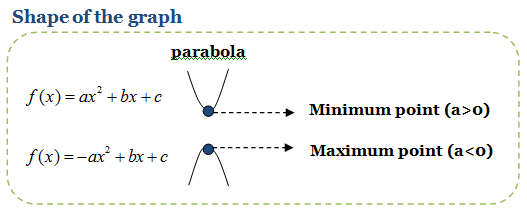2.1 牛顿法的优点：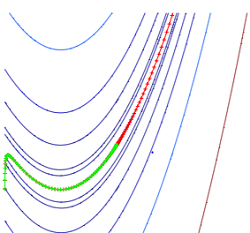2.2 牛顿法的缺点：

1.$$Hessian$$矩阵的计算难度非常的大。因此在高维度应用案例中，通常不会计算$$Hessian$$矩阵。因此牛顿法也产生了很多变种，主要的思想就是采用其他矩阵近似$$Hessian$$矩阵，降低计算复杂度。

2.牛顿法当$$Hessian$$矩阵为正定矩阵时，最小值才存在。牛顿法经常会因为$$Hessian$$矩阵不正定而发散(diverage)。因此 牛顿法并不是非常的稳定。

2.3 牛顿法求根公式与牛顿优化法之间的联系

3.参考文献：

1.gradient descent in a nutshell – towardsdatascience.com

2.Newton’s method in Optimization-wikipedia

4.Using Gradient Descent in Optimization and Learning – University Collage London

5.Difference between Gradient Descent method and Steepest Descent – stack exchange

6.In optimization, why is Newton’s method much faster than gradient descent?

1. 一个文科生无意中刷到这个博客，还挺喜欢的。

•whudj说道：

多谢关注！你是通过什么关键词搜到这个博客的，哈哈

2. 喜欢博主的网站，哈哈哈，请问是什么专业的呀？计算机吗？

•whudj说道：

感谢！目前职业是程序员，大学专业不是计算机专业，不过有很多相关课程。

3. 剛好在學convex optimization, 您的文章幫助很大，謝謝!

•whudj说道：

很高兴能对你有帮助！

4. 看到whu感觉是校友，很喜欢“高斯牛顿”、“梯度下降”这两篇文章，点赞！

•whudj说道：

谢谢校友，哈哈

•匿名说道：

刚好在做非线性拟合，这两篇文章太好了！！感谢！！